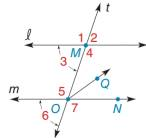Chapter 2.1, Problem 16EElementary Geometry For College St...

7th Edition
Alexander + 2 others
ISBN: 9781337614085

Solutions

Chapter
SectionElementary Geometry For College St...

7th Edition
Alexander + 2 others
ISBN: 9781337614085
Textbook Problem

Given: l ∥ m Transversal t m ∠ 1 = 4 x + 2 m ∠ 6 = 4 x − 2 Find: x and m ∠ 5Exercise 15, 16

To determine

To find:

The value of x and the measure of the given angle.

Explanation

The sum of angles that falls on the straight line is 180°.

Definition:

If there exists two parallel lines cut by a transversal then the pair of exterior angles on the same side of transversal is supplementary.

Given:

The given figure is,

lm cut by a transversal t.

The m1=4x+2 and m6=4x2.

Calculation:

Consider the given figure,

From the figure, lm with transversal t.

1 and 6 are exterior angles on the same side of transversal.

m1+m6=180°4x+2+4x2=180°8x=180°x=452

The value of x is 452.

Consider,

m1=4x+2…... (1)

Substitute 452 for x in equation (1)

Still sussing out bartleby?

Check out a sample textbook solution.

See a sample solution

The Solution to Your Study Problems

Bartleby provides explanations to thousands of textbook problems written by our experts, many with advanced degrees!

Get Started

In Exercises 2340, find the indicated limit. 34. limx1x3+12x3+2

Applied Calculus for the Managerial, Life, and Social Sciences: A Brief Approach

In Exercises 5-8, graph the given function or equation. 2x3y=12

Finite Mathematics and Applied Calculus (MindTap Course List)

f(x) = (x2 3)2 has points of inflection at x = _____. a) 0,3,3 b) 1, 1 c) 0, 1, 1 d) 3,3

Study Guide for Stewart's Single Variable Calculus: Early Transcendentals, 8th

In Exercises 1-8, determine which of the matrices are regular. 

Finite Mathematics for the Managerial, Life, and Social Sciences

True or False: is monotonic.

Study Guide for Stewart's Multivariable Calculus, 8th